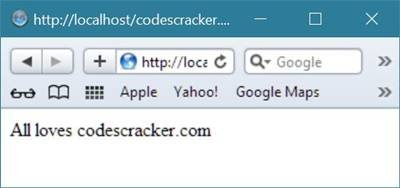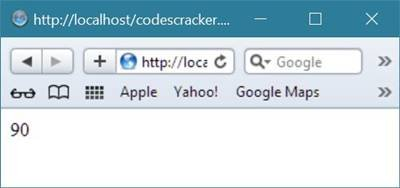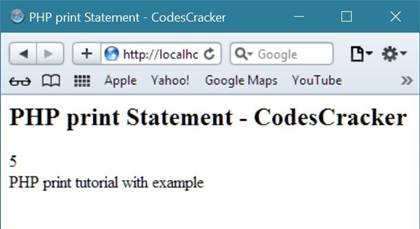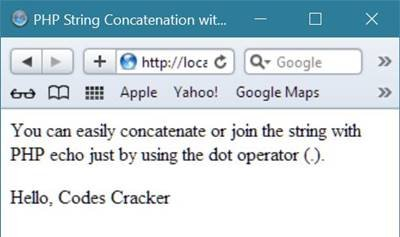# PHP echo and print

PHP echo keyword is approximately most usable keyword, used in almost each and every PHP program.

Both echo and print keyword is used in outputting anything in PHP.

Let's talk about how to output the value of a variable in PHP using echo keyword.

## PHP Output Value of Variable using echo

You can use PHP echo statement to output variable's value on the screen. Here is an example:

```<!DOCTYPE html>
<html>
<body>

<?php
\$txt = "codescracker.com";
echo "All loves \$txt";
?>

</body>
</html>```

It will display the following in the browser:The following example will produce the same output as the example above:

```<!DOCTYPE html>
<html>
<body>

<?php
\$txt = "codescracker.com";
echo "All loves " . \$txt;
?>

</body>
</html>```

It will display the same output as above:Look at the following example which will output the sum of two variables:

```<!DOCTYPE html>
<html>
<body>

<?php
\$x = 50;
\$y = 40;
echo \$x + \$y;
?>

</body>
</html>```

It will display the following:## PHP echo Example

Here is an example demonstrates how to use echo statement in PHP to output anything.

```<html>
<title>PHP echo Example - CodesCracker</title>
<body>
<h2>PHP echo Example - CodesCracker</h2>
<?php
# Example of PHP echo statement - CodesCracker
\$var1 = "Codes";
\$var2 = "Cracker";
\$var3 = 5;
\$var4 = 6;
echo "Value of first PHP variable = \$var1 <br/>";
echo "Value of second PHP variable = \$var2 <br/>";
echo "Value of third PHP variable = \$var3 <br/>";
echo "Value of fourth PHP variable = \$var4 <br/>";
?>
</body>
</html>```

Here is the sample output produced by the above PHP echo keyword/statement example code:## PHP print Example

PHP print statement is also used to output anything just like print.

Here is an example of print statement in PHP demonstrates how to use print statement/keyword in PHP to output anything in browser window.

```<html>
<title>PHP print Statement - CodesCracker</title>
<body>
<h2>PHP print Statement - CodesCracker</h2>
<?php
# PHP print statement Example - CodesCracker
\$num = 5;
\$var = "PHP print tutorial with example";
print \$num;
print "<br/>";
print \$var;
?>
</body>
</html>```

The above PHP print statement example code will produce the following output:## PHP echo and print Example

Here is an example uses both echo and print statement in the same PHP program.

```<html>
<title>PHP echo & print Example - CodesCracker</title>
<body>
<h3>PHP echo & print Example - CodesCracker</h3>
<?php
\$var1 = 25;
\$var2 = 26;
echo "<p>Sum of the two variable (PHP echo) = \$var1+\$var2</p>";
print "<p>Sum of the two variable (PHP print) = \$var1+\$var2</p>";
echo \$var1+\$var2;
echo "<br/>";
print \$var1+\$var2;
\$sum = \$var1+\$var2;
echo "<p>Sum (PHP echo) = \$sum</p>";
print "<p>Sum (PHP print) = \$sum</p>";
?>
</body>
</html>```

Here is the sample output of the above PHP echo and print statements example code:## PHP String Concatenation with echo

You can use dot operator (.) to concatenate any string in PHP using echo statement. Here is an example demonstrates how to concatenate two string in PHP using echo statement.

```<html>
<title>PHP String Concatenation with echo</title>
<body>
<p>You can easily concatenate or join the string with
PHP echo just by using the dot operator (.).</p>
<?php
# string concatenation example in PHP using echo - CodesCracker
\$name = "Codes";
\$surname = "Cracker";
echo "<p>Hello, ".\$name." ".\$surname."</p>";
?>
</body>
</html>```

Below is the sample output of the above string concatenation example code using echo statement in PHP:## PHP printf and sprintf Function

In PHP, you can use printf when you want to output formatted string using placeholders, whereas sprintf function returns the formatted string.

Let's take an example demonstrating the printf() and sprintf() function in PHP:

```<html>
<title>PHP printf and sprintf Example</title>
<body>
<?php
/*
You can use printf for outputting anything
directly whereas you can place the output material into any
PHP variable using the PHP sprintf function
*/
\$fullname = "CodesCracker";
printf("Hello sir! This is %s", \$fullname);
echo "<hr/>";
\$output_material = sprintf("Hello sir! This is %s", \$fullname);
echo \$output_material;
?>
</body>
</html>```

The above example code of printf() and sprintf() function in PHP will produce the following output:Tools
Calculator